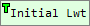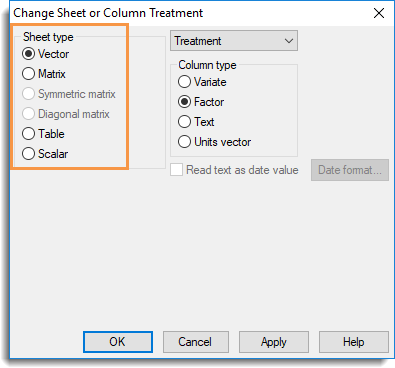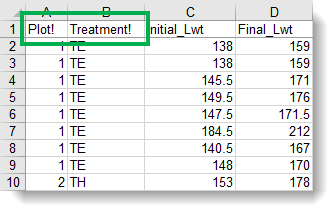1. Home
2. Converting Data Types (variates to factors, etc)

# Converting Data Types (variates to factors, etc)

Genstat saves your information as named data types. For example, a factor provides a classification into a number of distinct groups or classes. A variate is a list of numbers. A text can contain letters or a mixture of letters and numbers. The image below contains the initial and final live-weights of calves that were put in 12 paddocks to graze for two weeks, with each paddock containing one of 3 types of food. The column Plot is a factor that specifies the paddock number; Treatment is a factor that specifies the food; Initial Lwt and Final Lwt are both variates that record the calves’ weights.A factor is identified by an exclamation markappended to the column nameA text is identified by aappended to the column nameAfter importing data into Genstat you may want to change the data structure. For example, you may want to convert a spreadsheet column to a factor as it contains grouped data, or convert a factor to a text for use in a particular menu.

Convert a single column to a factor, variate or text

1. Right-click the column you want to convert and select one of the Convert to options.Convert multiple columns to a factor, variate or text

1. Hold down the Ctrl key and click the top of each column you want to convert. Now right-click and select one of the Convert to options.Convert an entire spreadsheet to another type of data structure (matrix, table, etc.)

1. Right-click anywhere in the spreadsheet and select Convert.
2. Select the Sheet type. The following restrictions apply to spreadsheet conversion:
• A vector spreadsheet containing text columns cannot be converted to any other type.
• Converting a spreadsheet to type scalar will preserve row 1 and discard all other rows.
• Spreadsheets can only be converted to diagonal or symmetric matrix type if they are square, i.e. have equal numbers of rows and columns.
• Converting a spreadsheet to type vector will produce columns of type variate.3. Additional fields may appear depending on which Sheet type you select. Click OK after setting additional options as described below.

Matrix or Table name
You can change the name of matrices or tables by typing the new name into this field.

Classify table with
When converting to a table, you can specify whether the new sheet has:

• 1 classifying factor – multiple one-way tables (one table per column)
• 2 classifying factors – a single two-way table with a factor indexing the columns.

Include table margins
When selected, if a table being converted to a vector or matrix sheet contains margins they will be included in the conversion, otherwise they will be removed.

Convert data before importing into Genstat

You can append certain characters to column names before importing so that they will open in Genstat as a particular data type. For example, to import a column as a factor append an exclamation mark ! to the end of the column name.

In this Excel spreadsheet the first two columns, Plot! and Treatment! both have an exclamation mark suffix. When this file is imported into Genstat these two columns will be interpreted as factors.The following markers can be added to column names.

 ! Interpret the column as a factor # Interpret the column as a variate \$ Interpret the column as a text :D Display the column as a date :T Display the column as a time :0…:9 Display the column with 0-9 decimal places :E Display the column in scientific format

The ! marker can be applied in conjunction with the Date :D or Time :T markers, so that !:D would indicate a factor with levels displayed as dates.

Updated on March 1, 2019